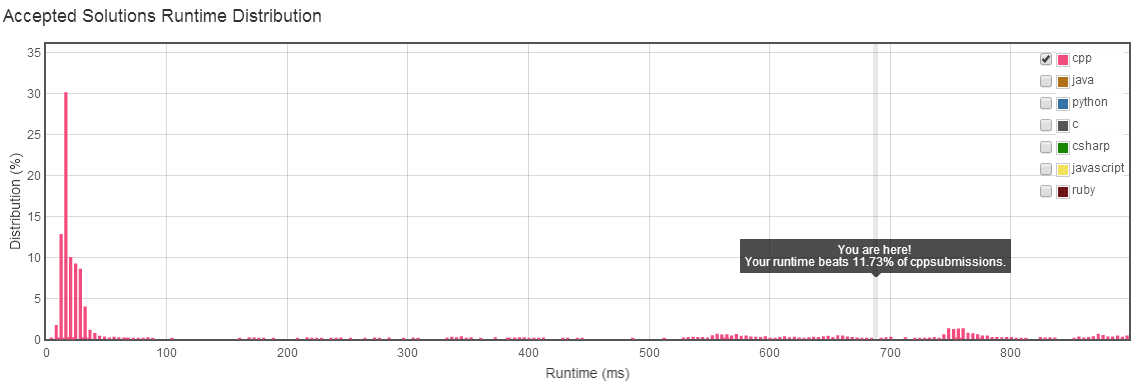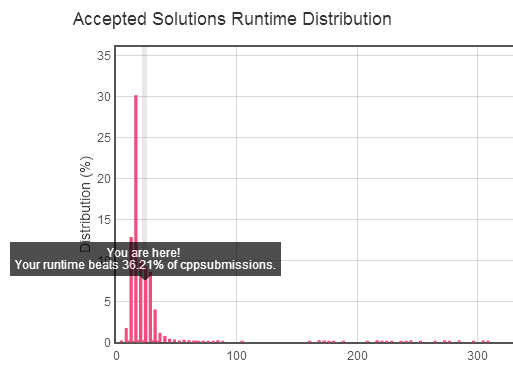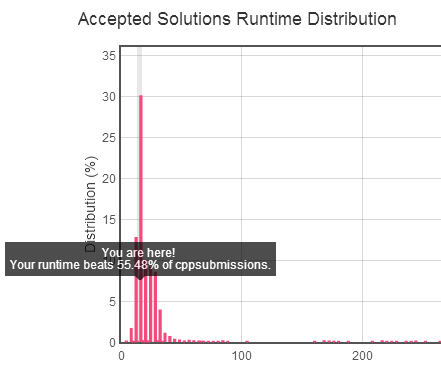## Two Sum#

https://leetcode.com/problems/two-sum/

## Solution 1#

``````class Solution {
public:
vector<int> twoSum(vector<int>& nums, int target) {
bool is_get = false;
int i = 0;
int j = 0;
for (; i < nums.size(); i++) {
for (j = i + 1; j < nums.size(); j++) {
if (nums[i] + nums[j] == target) {
is_get = true;
break;
}
}
if (is_get) {
break;
}
}
vector<int> v;
v.push_back(i);
v.push_back(j);
return v;
}
};
``````## Solution 2#

``````class Solution {
public:
vector<int> twoSum(vector<int>& nums, int target) {
map<int, int> map_subs;
vector<int> vec_reslut;
map<int, int>::iterator it;
for (size_t i = 0; i < nums.size(); i++) {
it = map_subs.find(nums[i]);
if(it != map_subs.end()) {
vec_reslut.push_back(it->second);
vec_reslut.push_back(i);
break;
}
map_subs[target - nums[i]] = i;
}

return vec_reslut;
}
``````## Solution 3#

``````class Solution {
public:
vector<int> twoSum(vector<int>& nums, int target) {
vector<int> vec_reslut;
unordered_map<int, int> map_subs;
unordered_map<int, int>::iterator it;
for (size_t i = 0; i < nums.size(); i++) {
it = map_subs.find(nums[i]);
if(it != map_subs.end()) {
vec_reslut.push_back(it->second);
vec_reslut.push_back(i);
break;
}
map_subs[target - nums[i]] = i;
}

return vec_reslut;
}
};
``````